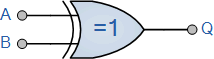# Exclusive-OR Gate Tutorial

## Exclusive-OR Gate Tutorial

Exclusive-OR Gate DefinitionBut there are two other types of digital logic gates which although they are not a basic gate in their own right as they are constructed by combining together other logic gates, their output Boolean function is important enough to be considered as complete logic gates. These two “hybrid” logic gates are called the Exclusive-OR (Ex-OR) Gate and its complement the Exclusive-NOR (Ex-NOR) Gate.

Previously, we saw that for a 2-input OR gate, if A = “1”, OR B = “1”, OR BOTH A + B = “1” then the output from the digital gate must also be at a logic level “1” and because of this, this type of logic gate is known as an Inclusive-OR function. The gate gets its name from the fact that it includes the case of Q = “1” when both A and B = “1”.

If however, an logic output “1” is obtained when ONLY A = “1” or when ONLY B = “1” but NOT both together at the same time, giving the binary inputs of “01” or “10”, then the output will be “1”. This type of gate is known as an Exclusive-OR function or more commonly an Ex-Or function for short. This is because its boolean expression excludes the “OR BOTH” case of Q = “1” when both A and B = “1”.

In other words the output of an Exclusive-OR gate ONLY goes “HIGH” when its two input terminals are at “DIFFERENT” logic levels with respect to each other.

An odd number of logic “1’s” on its inputs gives a logic “1” at the output. These two inputs can be at logic level “1” or at logic level “0” giving us the Boolean expression of:  Q = (A     B) = A.B + A.B

The Exclusive-OR Gate function, or Ex-OR for short, is achieved by combining standard logic gates together to form more complex gate functions that are used extensively in building arithmetic logic circuits, computational logic comparators and error detection circuits.

The two-input “Exclusive-OR” gate is basically a modulo two adder, since it gives the sum of two binary numbers and as a result are more complex in design than other basic types of logic gate. The truth table, logic symbol and implementation of a 2-input Exclusive-OR gate is shown below.

## The Digital Logic “Exclusive-OR” Gate

### 2-input Ex-OR Gate

 Symbol Truth Table2-input Ex-OR Gate B A Q 0 0 0 0 1 1 1 0 1 1 1 0 Boolean Expression Q = A     B A OR B but NOT BOTH gives Q

The truth table above shows that the output of an Exclusive-OR gate ONLY goes “HIGH” when both of its two input terminals are at “DIFFERENT” logic levels with respect to each other. If these two inputs, A and B are both at logic level “1” or both at logic level “0” the output is a “0” making the gate an “odd but not the even gate”.

This ability of the Exclusive-OR gate to compare two logic levels and produce an output value dependent upon the input condition is very useful in computational logic circuits as it gives us the following Boolean expression of:

Q = (A     B) = A.B + A.B

The logic function implemented by a 2-input Ex-OR is given as either: “A OR B but NOT both” will give an output at Q. In general, an Ex-OR gate will give an output value of logic “1” ONLY when there are an ODD number of 1’s on the inputs to the gate, if the two numbers are equal, the output is “0”.

Then an Ex-OR function with more than two inputs is called an “odd function” or modulo-2-sum (Mod-2-SUM), not an Ex-OR. This description can be expanded to apply to any number of individual inputs as shown below for a 3-input Ex-OR gate.

### 3-input Ex-OR Gate

Symbol Truth Table